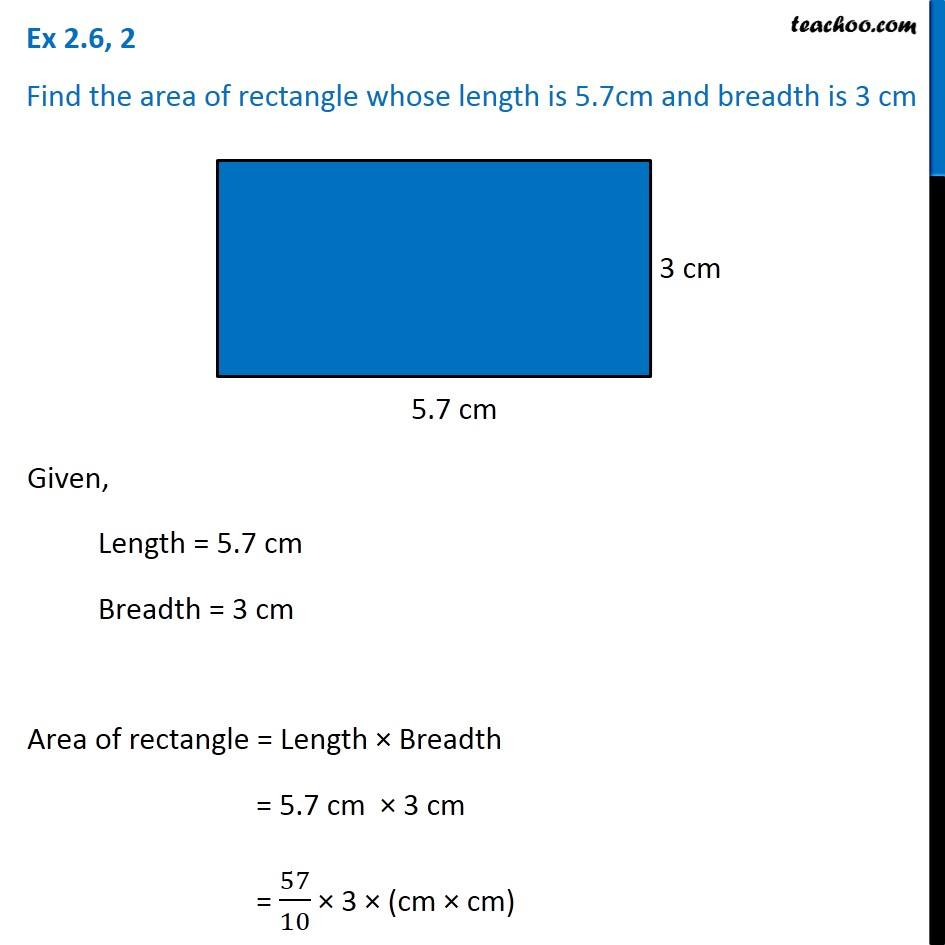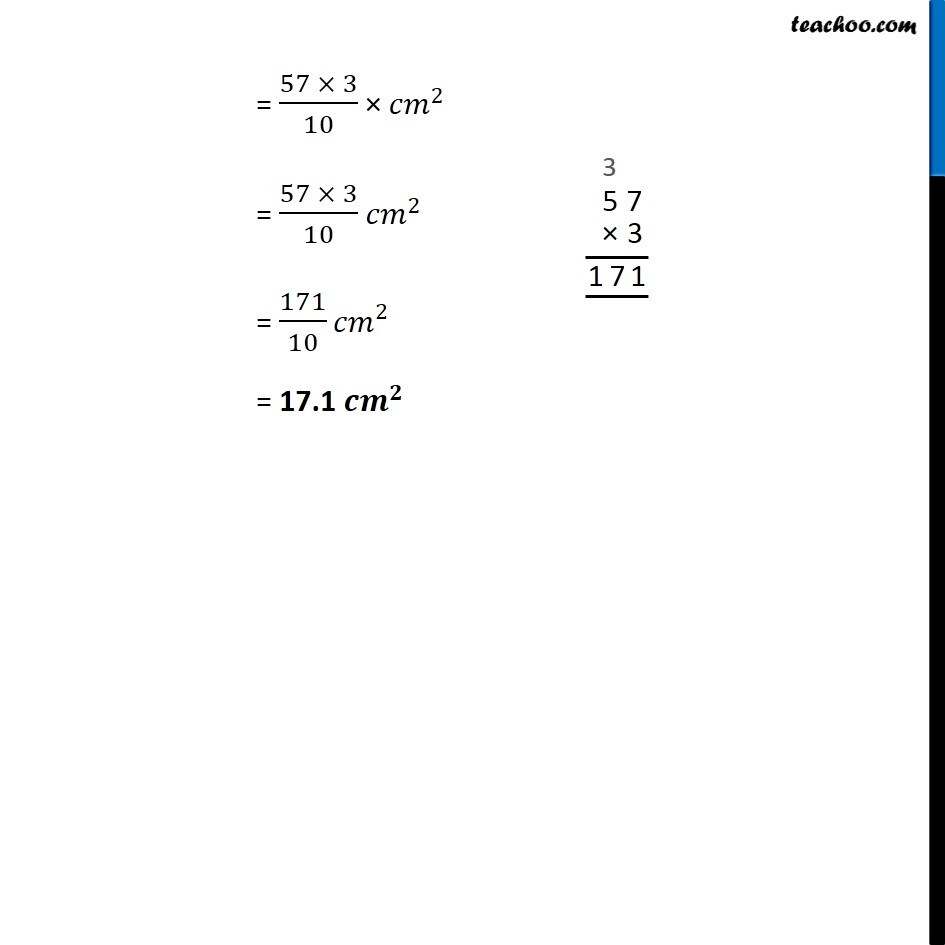Ex 2.4

Chapter 2 Class 7 Fractions and Decimals
Serial order wiseLearn in your speed, with individual attention - Teachoo Maths 1-on-1 Class

### Transcript

Ex 2.4, 2 Find the area of rectangle whose length is 5.7cm and breadth is 3 cmGiven, Length = 5.7 cm Breadth = 3 cm Area of rectangle = Length × Breadth = 5.7 cm × 3 cm = 57/10 × 3 × (cm × cm) = (57 × 3)/10 × 〖𝑐𝑚〗^2 = (57 × 3)/10 〖𝑐𝑚〗^2 = 171/10 〖𝑐𝑚〗^2 = 17.1 〖𝒄𝒎〗^𝟐 = (57 × 3)/10 × 〖𝑐𝑚〗^2 = (57 × 3)/10 〖𝑐𝑚〗^2 = 171/10 〖𝑐𝑚〗^2 = 17.1 〖𝒄𝒎〗^𝟐